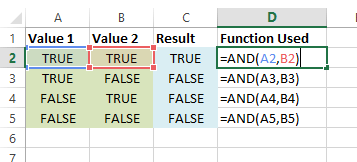This function will return TRUE if all arguments are TRUE and FALSE otherwise.

## Syntax

AND(Expression1, Expression2, Expression3, … )

• Expression1 (required) – This is an expression that can evaluate to either TRUE or FALSE.
• Expression2, Expression3 etc… (optional) – These are additional expressions you want to test.

## Example

In this example we test two expressions. As seen, the result is only true when both expressions evaluated are true.

``=AND(A2,B2)``